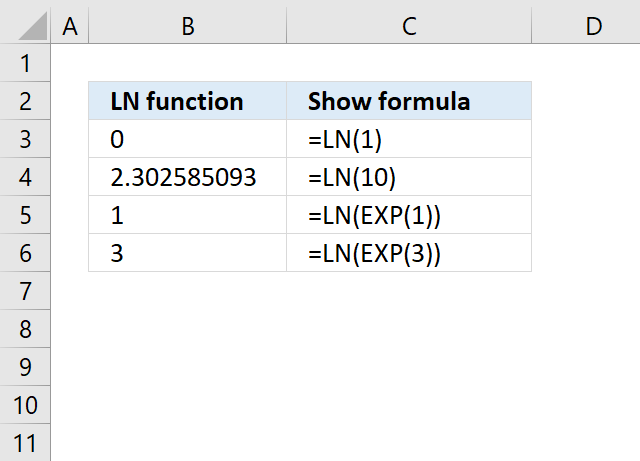Author: Oscar Cronquist Article last updated on April 18, 2018The LN function calculates the natural logarithm of a number. Natural logarithms are based on the constant e.

Formula in cell C3:

=LN(1)

LN(number)

### Arguments

 number Required. The positive numerical value for which you want the natural logarithm.

### Recommended article

How to use the EXP function

Returns e raised to the power of a number, e equals 2.71828182845904. Example, e^2 equals 7.389056099 Formula in cell C3: […]